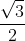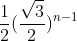## Tuesday, 1 February 2011

### Another Pretty Geometry Problem

Here is another nice problem from Math Central at the Univ of Regina. This one is related to the Log Spiral I recently wrote about.  It combines a little simple 30/60/90 right triangle geoemtry with a geometric series...A nice problem for letting the students create a geometric series (geometrically) rather than throwing out a formula. And at the end, a really cute solution by geometric transformations.

The unit circle is divided into twelve equal parts, and the twelve dividing points are joined to the circle's centre, producing twelve rays. Starting from one of the dividing points a segment is drawn perpendicular to the next ray in the clockwise sense; from the foot of this perpendicular another perpendicular segment is drawn to the next ray, and so on to the center of the circle (note the diagram only shows part of the total curve). What is the limit of the sum of the lengths of these segments?

The  classic solution approach is to see that each central angle is 30o and so the hypotenuse of each succeeding triangle is the cosine of ﻿30o  times the previous.  So the largest of the spiraling segments is opposite a 30o with a hypotenuse of one, and must have a lenth of 1/2.  Each succeeding length is the previous length times.    These form a geometric series with the nth  segment being.

The sum of an infinite sequence with a common ratio less than one is given byand in this case that becomes.

A really pretty solution was given that can be illustrated with some simple rotations..
If we look at just the first two triangles to make it simple...

And then rotate the second (30 degrees in this cae) about the connection with the previous segment we can align them in a vertical column...

This can be continued with each following segment and aligning the segments to produce a triangle like the one below.  [Students see this better, it seems, if you describe it as happening from the center out.  Note that we get a right triangle with the right angle at top of the first segment created by drawing a one unit segment parallel side from the origin.  The sum of the segments is now shown to be one leg of a triangle whose other leg is one.  So the lengths must sum to the tan(75o).  I call that pretty cool geometry.